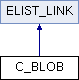Tesseract  3.02
C_BLOB Class Reference

`#include <stepblob.h>`

Inheritance diagram for C_BLOB:List of all members.

## Public Member Functions

C_BLOB ()
C_BLOB (C_OUTLINE_LIST *outline_list)
C_BLOB (C_OUTLINE *outline)
C_OUTLINE_LIST * out_list ()
TBOX bounding_box ()
inT32 area ()
inT32 perimeter ()
inT32 outer_area ()
inT32 count_transitions (inT32 threshold)
void move (const ICOORD vec)
void rotate (const FCOORD &rotation)
Pix * render ()
Pix * render_outline ()
void plot (ScrollView *window, ScrollView::Color blob_colour, ScrollView::Color child_colour)
C_BLOBoperator= (const C_BLOB &source)

## Static Public Member Functions

static C_BLOBFakeBlob (const TBOX &box)
static C_BLOBdeep_copy (const C_BLOB *src)

## Detailed Description

Definition at line 28 of file stepblob.h.

## Constructor & Destructor Documentation

 C_BLOB::C_BLOB ( ) ` [inline]`

Definition at line 31 of file stepblob.h.

```             {
}
```
 C_BLOB::C_BLOB ( C_OUTLINE_LIST * outline_list ) ` [explicit]`

Definition at line 143 of file stepblob.cpp.

```                {
C_OUTLINE *outline;            //current outline
C_OUTLINE_IT it = outline_list;//iterator

while (!it.empty ()) {         //grab the list
outline = it.extract ();     //get off the list
//put it in place
position_outline(outline, &outlines);
if (!it.empty ())
it.forward ();
}
it.set_to_list (&outlines);
for (it.mark_cycle_pt (); !it.cycled_list (); it.forward ()) {
outline = it.data ();
if (outline->turn_direction () < 0) {
outline->reverse ();
reverse_outline_list (outline->child ());
outline->set_flag (COUT_INVERSE, TRUE);
}
else {
outline->set_flag (COUT_INVERSE, FALSE);
}
}
}
```
 C_BLOB::C_BLOB ( C_OUTLINE * outline ) ` [explicit]`

Definition at line 172 of file stepblob.cpp.

```                                 {
C_OUTLINE_IT it(&outlines);
it.add_to_end(outline);
}
```

## Member Function Documentation

 inT32 C_BLOB::area ( )

Definition at line 211 of file stepblob.cpp.

```                   {  //area
C_OUTLINE *outline;            //current outline
C_OUTLINE_IT it = &outlines;   //outlines of blob
inT32 total;                   //total area

total = 0;
for (it.mark_cycle_pt (); !it.cycled_list (); it.forward ()) {
outline = it.data ();
total += outline->area ();
}
return total;
}
```
 TBOX C_BLOB::bounding_box ( )

Definition at line 192 of file stepblob.cpp.

```                          {  //bounding box
C_OUTLINE *outline;            //current outline
C_OUTLINE_IT it = &outlines;   //outlines of blob
TBOX box;                       //bounding box

for (it.mark_cycle_pt (); !it.cycled_list (); it.forward ()) {
outline = it.data ();
box += outline->bounding_box ();
}
return box;
}
```
 inT32 C_BLOB::count_transitions ( inT32 threshold )

Definition at line 271 of file stepblob.cpp.

```                                 {
C_OUTLINE *outline;            //current outline
C_OUTLINE_IT it = &outlines;   //outlines of blob
inT32 total;                   //total area

total = 0;
for (it.mark_cycle_pt (); !it.cycled_list (); it.forward ()) {
outline = it.data ();
total += outline->count_transitions (threshold);
}
return total;
}
```
 static C_BLOB* C_BLOB::deep_copy ( const C_BLOB * src ) ` [inline, static]`

Definition at line 74 of file stepblob.h.

```                                                {
C_BLOB* blob = new C_BLOB;
*blob = *src;
return blob;
}
```
 C_BLOB * C_BLOB::FakeBlob ( const TBOX & box ) ` [static]`

Definition at line 180 of file stepblob.cpp.

```                                        {
C_OUTLINE_LIST outlines;
C_OUTLINE::FakeOutline(box, &outlines);
return new C_BLOB(&outlines);
}
```
 void C_BLOB::move ( const ICOORD vec )

Definition at line 293 of file stepblob.cpp.

```                   {
C_OUTLINE_IT it(&outlines);  // iterator

for (it.mark_cycle_pt (); !it.cycled_list (); it.forward ())
it.data ()->move (vec);      // move each outline
}
```
 C_BLOB& C_BLOB::operator= ( const C_BLOB & source ) ` [inline]`

Definition at line 67 of file stepblob.h.

```                                              {
if (!outlines.empty ())
outlines.clear();
outlines.deep_copy(&source.outlines, &C_OUTLINE::deep_copy);
return *this;
}
```
 C_OUTLINE_LIST* C_BLOB::out_list ( ) ` [inline]`

Definition at line 42 of file stepblob.h.

```                               {  //get outline list
return &outlines;
}
```
 inT32 C_BLOB::outer_area ( )

Definition at line 250 of file stepblob.cpp.

```                         {  //area
C_OUTLINE *outline;            //current outline
C_OUTLINE_IT it = &outlines;   //outlines of blob
inT32 total;                   //total area

total = 0;
for (it.mark_cycle_pt (); !it.cycled_list (); it.forward ()) {
outline = it.data ();
total += outline->outer_area ();
}
return total;
}
```
 inT32 C_BLOB::perimeter ( )

Definition at line 230 of file stepblob.cpp.

```                        {
C_OUTLINE *outline;            // current outline
C_OUTLINE_IT it = &outlines;   // outlines of blob
inT32 total;                   // total perimeter

total = 0;
for (it.mark_cycle_pt(); !it.cycled_list(); it.forward()) {
outline = it.data();
total += outline->perimeter();
}
return total;
}
```
 void C_BLOB::plot ( ScrollView * window, ScrollView::Color blob_colour, ScrollView::Color child_colour )

Definition at line 376 of file stepblob.cpp.

```                   {
plot_outline_list(&outlines, window, blob_colour, child_colour);
}
```
 Pix * C_BLOB::render ( )

Definition at line 353 of file stepblob.cpp.

```                    {
TBOX box = bounding_box();
Pix* pix = pixCreate(box.width(), box.height(), 1);
render_outline_list(&outlines, box.left(), box.top(), pix);
return pix;
}
```
 Pix * C_BLOB::render_outline ( )

Definition at line 362 of file stepblob.cpp.

```                            {
TBOX box = bounding_box();
Pix* pix = pixCreate(box.width(), box.height(), 1);
render_outline_list_outline(&outlines, box.left(), box.top(), pix);
return pix;
}
```
 void C_BLOB::rotate ( const FCOORD & rotation )

Definition at line 328 of file stepblob.cpp.

```                                          {
RotateOutlineList(rotation, &outlines);
}
```

The documentation for this class was generated from the following files: